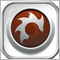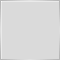# why -1 and i-- insead of935

for(int i = OrdersTotal()-1; i >= 0 ; i--)

IMO it seems to miss the latest order everytime wouldn't it ?

If OrdersTotal() were 4 lets say

(4-1)=3 so the (i) would be 3 and the selected order would be -1 of the OrdersTotal or even OrdersHistoryTotal(). This confuses me.

The documents suggest: OrdersHistoryTotal() and i++

This makes sense to me while -1 and i-- confuses me.
It seems even OrdersTotal() and i-- without the -1 makes more sense to me, but I am confused about the -1.22339

Agent86:

If OrdersTotal() were 4 lets say (4-1)=3 so the (i) would be 3

The documents suggest: OrdersHistoryTotal() and i++

1. If there are 4 orders, they are numbered 0, 1, 2, 3.
2. Get in the habit of counting down. Loops and Closing or Deleting Orders - MQL4 forum935

WHRoeder:
1. If there are 4 orders, they are numbered 0, 1, 2, 3.
2. Get in the habit of counting down. Loops and Closing or Deleting Orders - MQL4 forum
OH WAIT, OrdersTotal() and OrdersTotalHistory() are in type. I didn't see in any documentation where 0 being the first as you would normally with [] index types

So your saying 0 would actually  be an order ? Similar to  index types are treated ?
Perhaps this is where I am confused.

Thanks ALOT for the responses1868

`for(int i = OrdersTotal()-1; i >= 0 ; i--)`
Ever thought why i >= 0; ? Get that figured out and welcome to the world of loops.Moderator
2312

Agent86:
So your saying 0 would actually  be an order ? Similar to  index types are treated ?
Perhaps this is where I am confused.

Yes, 0 is an order.935

deysmacro:
Ever thought why i >= 0; ? Get that figured out and welcome to the world of loops.
Why do I see frequent use of if(OrdersTotal() > 0) ?
Wouldn't this be missing the open order 0 all the time ?

Also, why does OrdersTotal() return 0 when there are NO market or pending orders open ?

Print("OrdersTotal ",OrdersTotal());  /// prints 1's and 0's for my EA that places only 1 trade at a time ?Moderator
2312

Try not to confuse how many orders there are with what the order indices are. OrdersTotal() does not return an index.

If OrdersTotal() return 0, there are no orders. Any attempt to retrieve an index is out of range.

If OrdersTotal() returns 1, there is 1 order and its index is 0.

If OrdersTotal() returns 2, there are 2 orders and their indices are 0 and 1.

And so forth935

honest_knave:

Try not to confuse how many orders there are with what the order indices are. OrdersTotal() does not return an index.

If OrdersTotal() return 0, there are no orders. Any attempt to retrieve an index is out of range.

If OrdersTotal() returns 1, there is 1 order and its index is 0.

If OrdersTotal() returns 2, there are 2 orders and their indices are 0 and 1.

And so forth

OK now I'm really confused
I mean why not just i=OrdersTotal() ?

So for this loop below
for(int i = OrdersTotal()-1; i >= 0 ; i--)

For example:

If OrdersTotal() were (2) for example:
And the loop says OrderTotal(2)-1. Isn't this order number 1 with an index of 

Doesn't this always skip the last order number 2 with index  ?

If that is not how works, then I can't seem to comprehend how it selects the last order in OrdersTotal() when using -1; or why that is preferred over this ----  for( i = OrderstTotal(); i>=0; i--) ?

Thanks for the responseModerator
2312

Agent86:

OK now I'm really confused
I mean why not just i=OrdersTotal() ?

i for index

There is never an order index == OrdersTotal().

The reason is because we count from 1 upwards with the number of orders... 1-2-3-4-5-6-7

But we count from 0 upwards with indices of the orders. 0-1-2-3-4-5-6 Does it help if you think of the indices as being labelled A-B-C-D-E-F-G rather than 0-1-2-3-4-5-6?

You are comparing apples (the number of orders) with pears (the index of order numbers).17271

Agent86:

OK now I'm really confused
I mean why not just i=OrdersTotal() ?

It's an array . . .  if you have an array holding your 5 open orders they are in the array in positions 0, 1, 2, 3 and 4   and  OrdersTotal() = 522339

Agent86: OK now I'm really confused I mean why not just i=OrdersTotal() ?
As honest_knave and I both tried to say, you are confusing the position numbers with the count.
```int nOpenOrders      = OrdersTotal();    // count     = 4
int iHighestPosition = nOpenORders - 1;  // highest   = 3
int iLowestPosition  = 0;                // lowest    = 0
// positions = 0, 1, 2, 3 : total of 4
for(int iPos = iHighestPosition; iPos >= iLowestPosition; --iPos){ // ALL open orders```